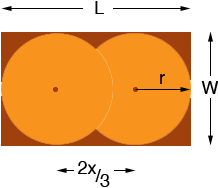SEARCH HOMEMath Central Quandaries & QueriesQuestion from Alex, a parent: One side of a rectangle is 10 and the other is x. Two circles with equal radii are inscribed in the rectangle and like a Venn Diagram, the circles overlap. Each circle touches the top and bottom of the rectangle and the left circle touches the left side of the rectangle and the right circle touches the right side of the triangle. The distance between the centre of each circle is 2x/3. Find xHi Alex,

It's not clear from the question which is larger, x or 10. I am going to think of the rectangle having length L and width W with L > W so you will have to this twice, once for L =10,W = x and again for L = x and W = 10. Let be the radius of the circle.Find r in terms of L and x. But W = 2r. Solve for x.

PennyMath Central is supported by the University of Regina and The Pacific Institute for the Mathematical Sciences.